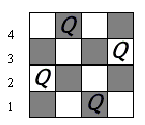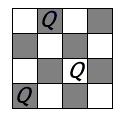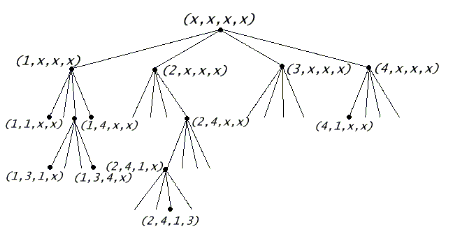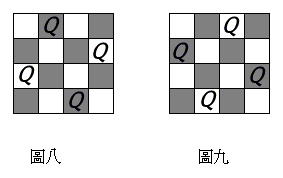```

8個皇后排在8乘以8的棋盤上，卻不使彼此攻伐的排法有幾種？數學家高斯猜測有96個解，圖一圖二

1,4,2,X)不管怎麼放，都不可能符合上開條件。下列樹狀圖每個節點表示棋盤上放皇后的一/**
* Program Name: Queen.java
* Purpose: 找N皇后問題有幾組解
* Author: Shiuh-Sheng Yu
* Date: 2003/05/20
*/
import java.io.*;
public class Queen {
private int[] board, directions;
private int size, numSolutions;
public static final int QUEEN = 0x01000000;
public static void main(String[] argv) {
int size, c;
for (;;) {
StringBuffer sb = new StringBuffer();
System.out.print("Please input the Queen size: ");
for (;;) {
try {
c = System.in.read();
if (!(c>='0' && c<='9')) {
if (sb.length()>0) break;
continue;
}
} catch(IOException ex) {
return;
}
sb.append((char)c);
}
try {
size = Integer.parseInt(sb.toString());
} catch(NumberFormatException ex) {
continue;
}
if (size==0) break;
Queen x = new Queen(size);
x.arrange();
System.out.println("The "+size+" Queen has "+x.getSolutionNum()+" solutions");
}
}
public Queen(int size) {
this.size = size;
directions = new int;
directions = size+1;
directions = size+2;
directions = size+3;
board = new int[(size+2)*(size+2)];
int lastLine = (size+2)*(size+1);
// 設定盤面上的哨兵
for (int i=0; i< size+2; i++) { // 上下兩排
board[i] = -1;
board[lastLine+i] = -1;
}
for (int i=1; i<=size; i++) { // 左右兩排
board[i*(size+2)] = -1;
board[i*(size+2)+size+1] = -1;
}
}
public int getSolutionNum() {
return numSolutions;
}
public void arrange() {
arrange(1);
}
/**
* 嘗試放到第row列
*/
private void arrange(int row) {
for (int i=1; i <= size; i++) { // 檢查此列上的每一行
int puton = (size+2)*row+i;
if (board[puton] == 0) { // 不在任何皇后範圍內
if (row==size) { // 已經到最後一個row, 此為一種解
numSolutions++;
} else {
board[puton] = QUEEN; // 放上皇后
for (int j=0; j<3; j++) { // 設定此皇后的勢力範圍
for (int k=puton+directions[j]; board[k]>=0; k+=directions[j]) {
board[k]++;
}
}
arrange(row+1);
board[puton] = 0; // 移除皇后
for (int j=0; j<3; j++) { // 移除此皇后的勢力範圍
for (int k=puton+directions[j]; board[k]>=0; k+=directions[j]) {
board[k]--;
}
}
}
}
}
}
}

```